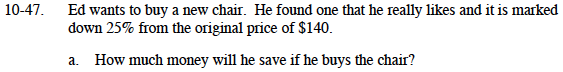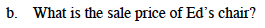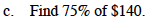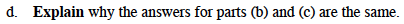### Home > MC1 > Chapter 10 > Lesson 10.1.4 > Problem10-47

10-47.Think of 25% as 2(10%) + 0.5(10%).
10% of $140 is$14

2($14) + 0.5($14) = $20 +$7 = $35 off$140 − \$35 = ?Refer to part (a) for help on how to do this part.100% − 25% = 75%
full price − discount = sale price